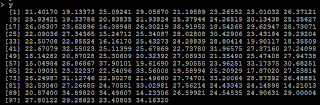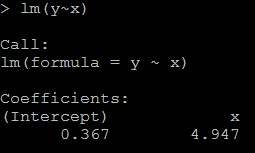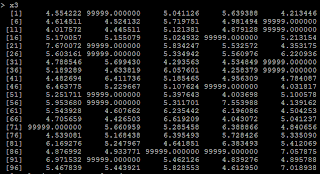## Monday, April 1, 2013

### The possible reason getting irregular coefficient(like we think it is positive, but it shows negetive)

Sometimes, we will get irregular coefficient: consider sell_amount vs sell_price, we should expect a negative coefficient because as the price increases, the selling amount should become less from common sense. But sometimes it may give a positive coefficient.

The possible reason is we put high correlated variables in the predictors: suppose we have both sell_price and product_price in the model, and we know product_price is highly correlated to sell_price. An example is shown below in example 1.

Another  possible reason is we recode the missing data: if missing data is at the left, but we recode it to the right(like if x<0 we recode x=99999 which is a very big number).

Example 1:

`# set up random number seed`
`set.seed(1000)`
` `
`# generate 100 x with x ~ N(5,1)`
`x=rnorm(100,5,1)`
` `
``
` `
`# Y=5*X`
`y=5*x+rnorm(100,0,1)`
` `
``
` `
`# X1=100*X`
`x1=x*100+rnorm(100,0,.2)`
` `
``
` `
` `
`1)it is reasonable that the regression coefficient is 4.947(while the true value is 5). `
`#relation between x and y`
`lm(y~x)`
` `
``
` `
`2) because x1=100*x, the coefficient for x1 should be 1/100 of above, as below:`
`# relation between x1 and y`
`lm(y~x1)`
` `
``
` `
`3) but if we put both x and x1 in the model, then we could not get the positive coefficient for both x and x1. This is because of multicollinearity. `
`# put both x and x1 as the predictor`
`lm(y~x+x1)`
` `
``
` `
` `

Example 2:

`4) another condition is from we recode missing data. Suppose if x<=4 is missing, and we recode missing as 99999.`
`# if x<=4, treat it as 99999`
`x3=ifelse(x>4,x,99999)`
` `
``
` `
`5) Then the regression coefficient is negative because of the 99999.`
`# relation between x3 and y`
`lm(y~x3)`
` `

The recode issue can be treated as a special case of non-linear relation.

1.2.3.